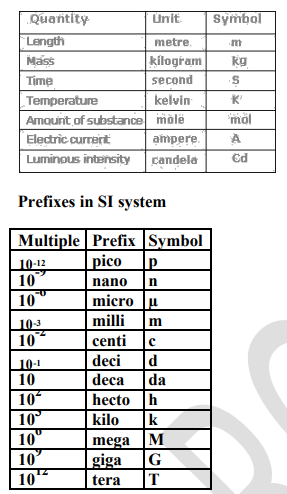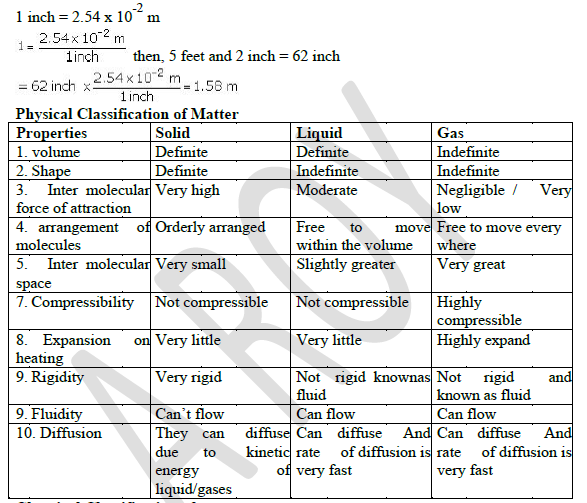# CBSE Class 11 Chemistry Revision Notes Set F

Download CBSE Class 11 Chemistry Revision Notes Set F in PDF format. All Revision notes for Class 11 Chemistry have been designed as per the latest syllabus and updated chapters given in your textbook for Chemistry in Standard 11. Our teachers have designed these concept notes for the benefit of Grade 11 students. You should use these chapter wise notes for revision on daily basis. These study notes can also be used for learning each chapter and its important and difficult topics or revision just before your exams to help you get better scores in upcoming examinations, You can also use Printable notes for Class 11 Chemistry for faster revision of difficult topics and get higher rank. After reading these notes also refer to MCQ questions for Class 11 Chemistry given our website

## Class 11 Chemistry Revision Notes

Class 11 Chemistry students should refer to the following concepts and notes for Chemistry in standard 11. These exam notes for Grade 11 Chemistry will be very useful for upcoming class tests and examinations and help you to score good marks

### Notes Class 11 Chemistry

UNIT 1

SOME BASIC CONCEPTS OF CHEMISTRY

Chemistry: Chemistry is the branch of science that deals with the composition, structure and properties of matter. Chemistry is called the science of atoms and molecule

Branches of Chemistry

•Organic Chemistry -This branch deals with study of carbon compounds especially hydrocarbons and their derivatives.

•Inorganic Chemistry-This branch deals with the study of compounds of all other elements except carbon. It largely concerns itself with the study of minerals found in the Earth's crust.

•Physical Chemistry-The explanation of fundamental principles governing various chemical phenomena is the main concern of this branch. It is basically concerned with laws and theories of the different branches of chemistry.

•Industrial Chemistry-The chemistry involved in industrial processes is studied under this branch.

•Analytical Chemistry-This branch deals with the qualitative and quantitative analysis of various substances.

•Biochemistry-This branch deals with the chemical changes going on in the bodies of living organisms; plants and animals.

•Nuclear Chemistry-Nuclear reactions, such as nuclear fission, nuclear fusion, transmutation processes etc. are studied under this branch.

PROPERTIES OF MATTER AND THEIR MEASUREMENT-Every substance has unique or characteristic properties. These properties can be classified into two categories – physical properties and chemical properties.

Physical properties are those properties which can be measured or observed without changing the identity or the composition of the substance. E.g. colour, odour, melting point, boiling point, density etc.

The measurement or observation of chemical properties requires a chemical change to occur. e.g. Burning of Mg-ribbon in air

Chemical properties are characteristic reactions of different substances; these include acidity or basicity, combustibility etc.Many properties of matter such as length, area, volume, etc., are quantitative in nature.

Metric System was based on the decimal system.

The International System of Units (SI)

The International System of Units (in French Le Systeme International d’Unites– abbreviated as SI) was established by the 11th General Conference on Weights and Measures (CGPM from ConferenceGenerale des Poids at Measures). The SI system has seven base units.Mass and Weight-- Mass of a substance is the amount of matter present in it while weight is the force exerted by gravity on an object. The mass of a substance is constant whereas its weight may vary from one place to another due to change in gravity. The mass of a substance can be determined very accurately by using an analytical balance
Volume-- Volume has the units of (length)3. So volume has units of m3 or cm3 or dm3.A common unit, litre (L) is not an SI unit, is used for measurement of volume of liquids. 1 L = 1000 mL, 1000 cm3 = 1 dm3

Density: Density of a substance is its amount of mass per unit volume.SI unit of density = SI unit of mass/SI unit of volume = kg/m3 or kg m–3This unit is quite large and a chemist often expresses density in g cm–3.
Temperature--There are three common scales to measure temperature — °C (degree celsius), °F (degree Fahrenheit) and K (kelvin). Here, K is the SI unit.
K = °C + 273.15
0F=9/5(°C)+32

Note—Temperature below 0 °C (i.e. negative values) are possible in Celsius scale but in Kelvin scale, negative temperature is not possible.
Scientific Notation
In which any number can be represented in the form N × 10n (Where n is an exponent having positive or negative values and N can vary between 1 to 10).
e.g. We can write 232.508 as 2.32508 x102 in scientific notation. Similarly, 0.00016 can be written as 1.6 x 10–4.
Precision refers to the closeness of various measurements for the same quantity. Accuracy is the agreement of a particular value to the true value of the result

Significant Figures
The reliability of a measurement is indicated by the number of digits used to represent it. To express it more accuratelywe express it with digits that are known with certainty. These are called as Significant figures. They contain all thecertain digits plus one doubtful digit in a number.

Rules for Determining the Number of Significant Figures

• All non-zero digits are significant. For example, 6.9 has two significant figures, while 2.16 has three significantfigures. The decimal place does not determine the number of significant figures.
• A zero becomes significant in case it comes in between non zero numbers. For example, 2.003 has four significantfigures, 4.02 has three significant figures.
• Zeros at the beginning of a number are not significant. For example, 0.002 has one significant figure while 0.0045has two significant figures.
• All zeros placed to the right of a number are significant. For example, 16.0 has three significant figures, while 16.00has four significant figures. Zeros at the end of a number without decimal point are ambiguous.
• In exponential notations, the numerical portion represents the number of significant figures. For example, 0.00045 isexpressed as 4.5 x 10-4 in terms of scientific notations. The number of significant figures in this number is 2, while inAvogadro's number (6.023 x 1023) it is four.
• The decimal point does not count towards the number of significant figures. For example, the number 345601 has sixsignificant figures but can be written in different ways, as 345.601 or 0.345601 or 3.45601 all having same number ofsignificant figures.

Retention of Significant Figures - Rounding off Figures
The rounding off procedure is applied to retain the required number of significant figures.

1. If the digit coming after the desired number of significant figures happens to be more than 5, the precedingsignificant figure is increased by one, 4.317 is rounded off to 4.32.

2. If the digit involved is less than 5, it is neglected and the preceding significant figure remains unchanged, 4.312 isrounded off to 4.31.

3. If the digit happens to be 5, the last mentioned or preceding significant figure is increased by one only in case ithappens to be odd. In case of even figure, the preceding digit remains unchanged. 8.375 is rounded off to 8.38 while8.365 is rounded off to 8.36.
Dimensional Analysis During calculations generally there is a need to convert units from one system to other. This is called factor label method or unit factor method or dimensional analysis.
For example- 5 feet and 2 inches (height of an Indian female) is to converted in SI unit
1 inch = 2.54 x 10-2 mChemical Classification of matter---Elements
An element is the simplest form of matter that cannot be split into simpler substances or built from simpler substances by any ordinary chemical or physical method. There are 114 elements known to us, out of which 92 are naturally occurring while the rest have been prepared artificially.
Elements are further classified into metals, non-metals and metalloids.

Compounds
A compound is a pure substance made up of two or more elements combined in a definite proportion by mass, which could be split by suitable chemical methods.

Characteristics of compound
• Compounds always contain a definite proportion of the same elements by mass.
• The properties of compounds are totally different from the elements from which they are formed.
• Compounds are homogeneous.
• Compounds are broadly classified into inorganic and organic compounds. Inorganic compounds are those, which areobtained from non-living sources such as minerals. For example, common salt, marble and limestone. Organiccompounds are those, which occur in living sources such as plants and animals. They all contain carbon. Commonorganic compounds are oils, wax, fats etc.

Mixtures
A mixture is a combination of two or more elements or compounds in any proportion so that the components do not lose their identity. Air is an example of a mixture Mixtures are of two types, homogeneous and heterogeneous.
Homogeneous mixtures have the same composition throughout the sample. The components of such mixtures cannot be seen under a powerful microscope. They are also called solutions. Examples of homogeneous mixtures are air, seawater, gasoline, brass etc.
Heterogeneous mixtures consist of two or more parts (phases), which have different compositions. These mixtures have visible boundaries of separation between the different constituents and can be seen with the naked eye e.g., sand and salt, chalk powder in water etc.